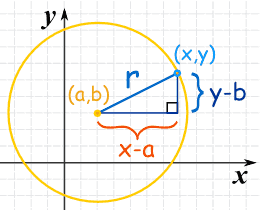## Equation of Circle

The equation of circle is:$\boxed{(x-a)^2+(y-b)^2=r^2}$

Centre: (a,b)

## Memory Tip

• The equation is actually Pythagoras’ Theorem !!!
• Please take note of the minus sign “(x-a), (y-b)”## Finding equation of circle (A Maths) 8 mark Question!

Question:

Find the equation of the circle which passes through$A(8,1)$ and$B(7,0)$ and has, for its tangent at$B$, the line$3x-4y-21=0$.

Solution:

Recall that the equation of a circle is$(x-a)^2+(y-b)^2=r^2$, where$(a,b)$ is the centre of the circle, and$r$ is the radius of the circle.

Substituting$A(8,1)$ into the equation, we get:$\boxed{(8-a)^2+(1-b)^2=r^2}$ — Eqn (1)

Substituting$B(7,0)$, we get:$\boxed{(7-a)^2+(0-b)^2=r^2}$ — Eqn (2)

Equating Eqn (1) and Eqn (2), we get$64-16a+a^2+1-2b+b^2=49-14a+a^2+b^2$ which reduces to$\boxed{b=8-a}$ — Eqn (3)

after simplification.

Now, we rewrite the equation of the tangent as$\displaystyle y=\frac{3}{4}x-\frac{21}{4}$ (make y the subject)

Hence, the gradient of the normal is$\displaystyle\frac{-1}{\frac{3}{4}}=-\frac{4}{3}$

Let the equation of the normal be$\displaystyle y=-\frac{4}{3}x+c$

Substitute in$B(7,0)$ we get$\displaystyle 0=-\frac{4}{3}(7)+c$

Hence$\displaystyle c=\frac{28}{3}$

Thus equation of normal is$\displaystyle \boxed{y=-\frac{4}{3}x+\frac{28}{3}}$

Since the normal will pass through the centre$(a,b)$ we have$\boxed{b=-\frac{4}{3}a+\frac{28}{3}}$ — Eqn (4)

Finally, we equate Eqn (3) and Eqn (4),$\displaystyle 8-a=-\frac{4}{3}a+\frac{28}{3}$$\displaystyle \frac{1}{3}a=\frac{4}{3}$$a=4$$b=8-a=4$

Substituting back into Eqn (1), we get$r=5$

Hence the equation of the circle is:$\displaystyle\boxed{(x-4)^2+(y-4)^2=5^2}$